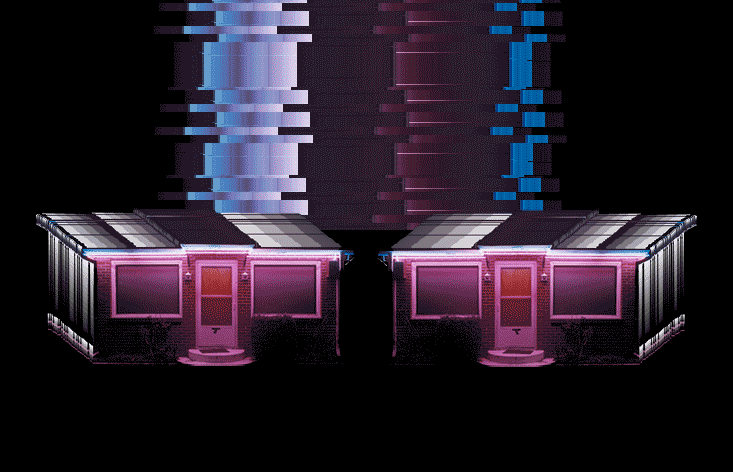⟑ ᖇ ᑕ ᕼ ᓮ V ᕮ

𝒸𝒽𝓇ℴ𝓃ℴ𝓁ℴℊ𝒾𝒸𝒶𝓁 ⑅ ⟑ ᖇ ᑕ ᕼ ᓮ V ᕮ

oblivion on the rocks
𓊍 2008-04-30 14:44:00

the shifting of planets and you
𓊍 2008-04-28 12:45:00

let freedom ring
𓊍 2008-04-22 16:46:00

goodbye to romance
𓊍 2008-04-18 14:52:00

vampire valley pipe dreams
𓊍 2008-04-14 15:53:00

melt mirage melt
𓊍 2008-04-07 22:38:00

white lightening flesh. black velvet bone.
𓊍 2008-04-04 12:13:00

4

the guiding light
𓊍 2008-03-31 22:28:00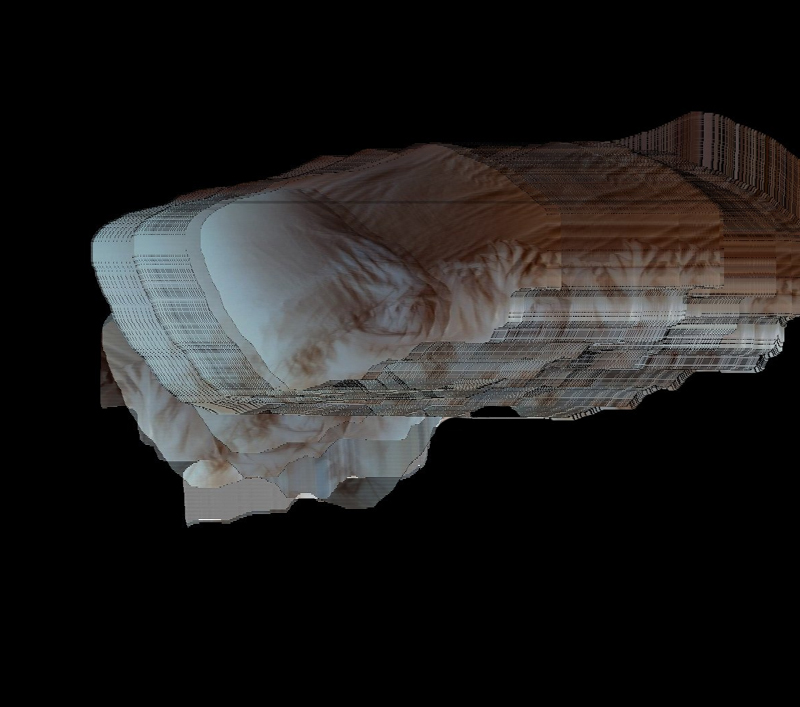we are the children of the sun
𓊍 2008-03-24 22:19:00

in a miami heartbeat
𓊍 2008-03-19 22:22:00

and ever and ever
𓊍 2008-03-13 21:21:00

there’s a path you take and a path untaken
𓊍 2008-03-10 00:21:00

the fixtures of fate
𓊍 2008-03-06 23:05:00

nights under the neon lights
𓊍 2008-03-03 23:12:00

we gathered for warmth around the computerized video camp fires of the city
𓊍 2008-02-27 20:35:00

shine electric left behind
𓊍 2008-02-24 22:41:00

round these parts
𓊍 2008-02-21 16:32:00

subdivisions of the forest line
𓊍 2008-02-12 01:08:00

(can't stop the) vision of love
𓊍 2008-02-04 16:16:00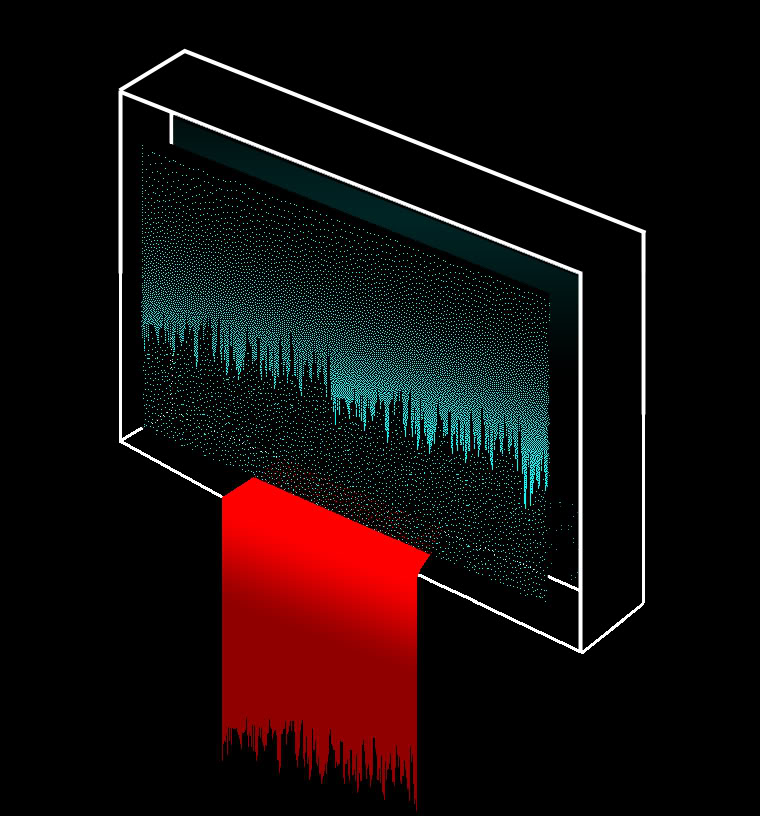vision of love
𓊍 2008-02-03 14:28:00

the fire in your eyes keeps me alive
𓊍 2008-01-31 16:19:00

dark alley warm car
𓊍 2008-01-29 19:26:00

i've been meaning to tell you
𓊍 2008-01-29 14:17:00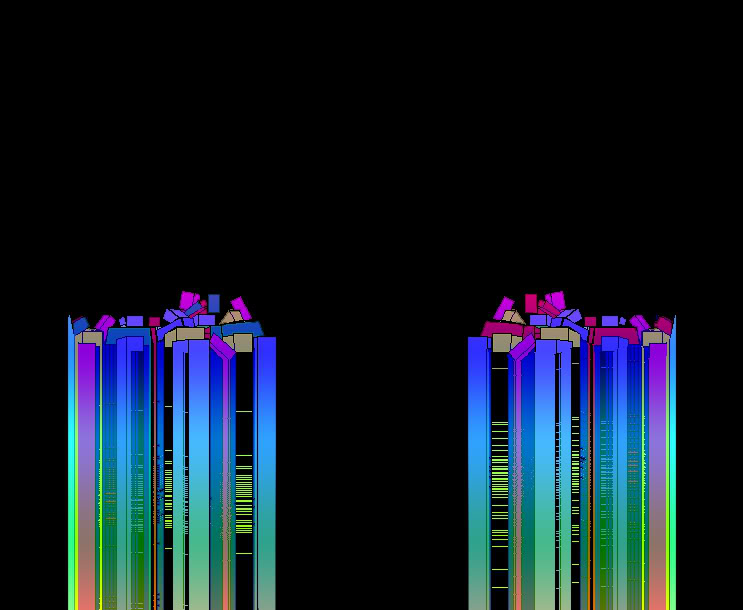a neutron dance for a neutron fan
𓊍 2008-01-29 14:07:00

perished parrish, secure alone
𓊍 2008-01-24 13:37:00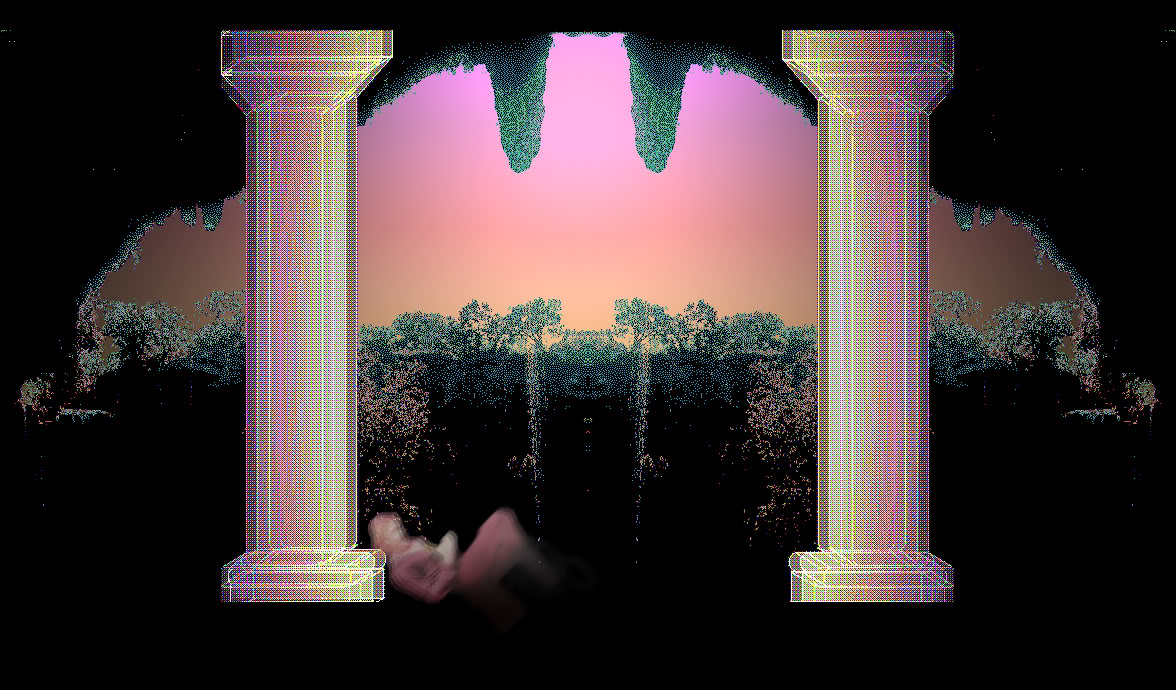𓊍 2008-01-21 21:15:00

fight for flight goodbye my dear
𓊍 2008-01-15 19:10:00

sustenance
𓊍 2008-01-04 22:22:00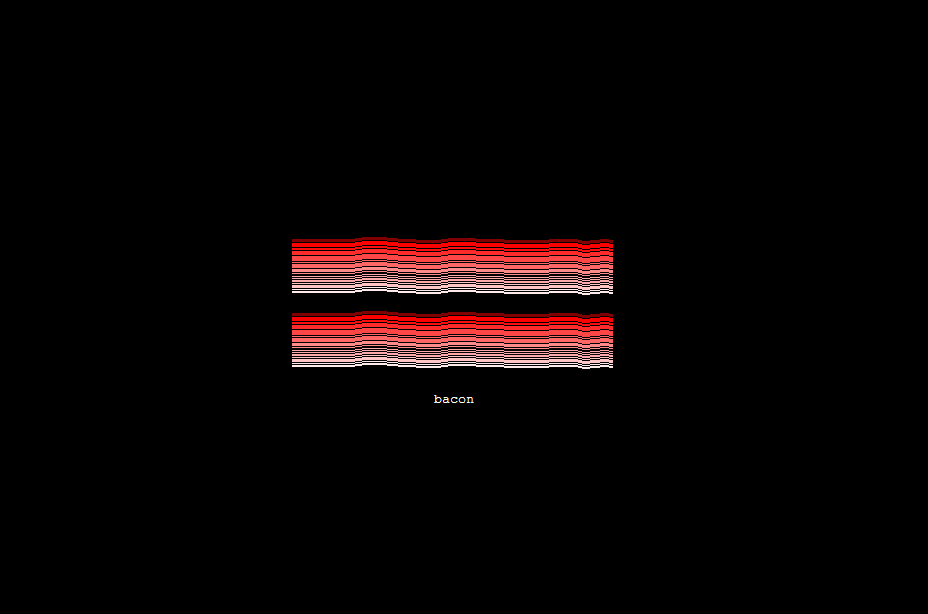the hills are alive but the dream is dead
𓊍 2008-01-03 23:01:00

come and be real for us
𓊍 2007-12-25 23:33:00

too much beauty sleep for sleeping beauty
𓊍 2007-12-16 23:18:00

sound surrounds the icy waters underground
𓊍 2007-12-14 20:30:00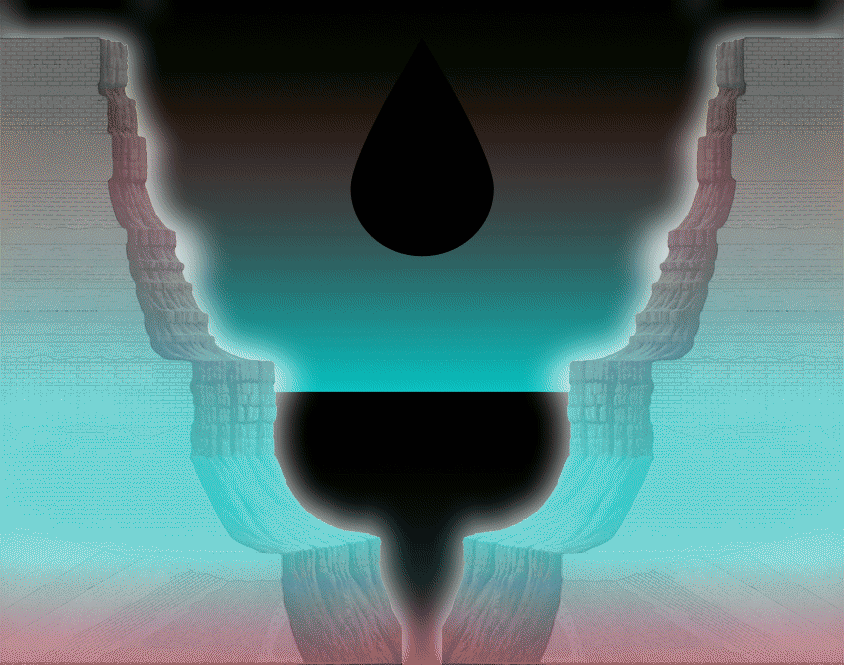loop dreams
𓊍 2007-12-04 15:03:00

there aint no way to hide your melting skies
𓊍 2007-12-03 23:51:00

scene of the silver studded jean dream
𓊍 2007-11-26 22:31:00

come out destruction
𓊍 2007-11-20 13:37:00

extreme echoing
𓊍 2007-11-14 23:31:00

in search of the next level
𓊍 2007-11-13 22:44:00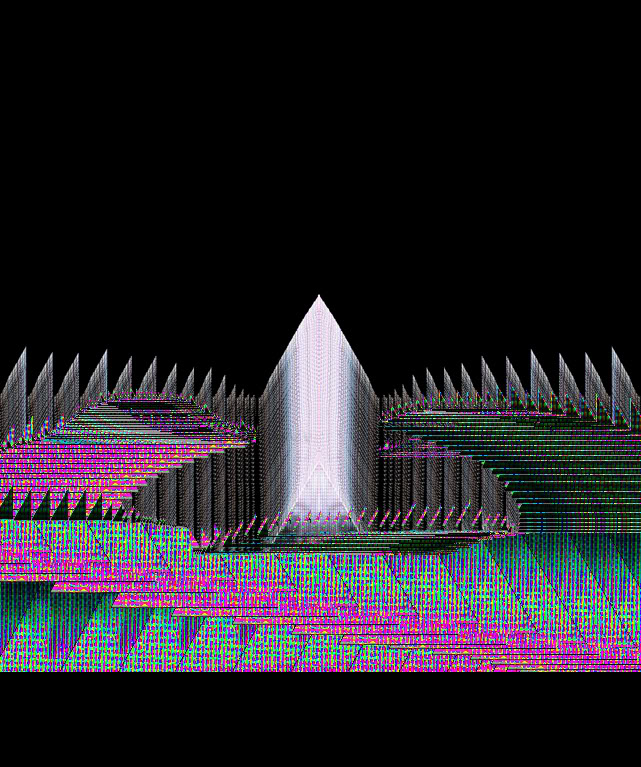scope of the skeletal scape
𓊍 2007-11-06 22:41:00

freedom glam on the permafrost
𓊍 2007-10-31 12:31:00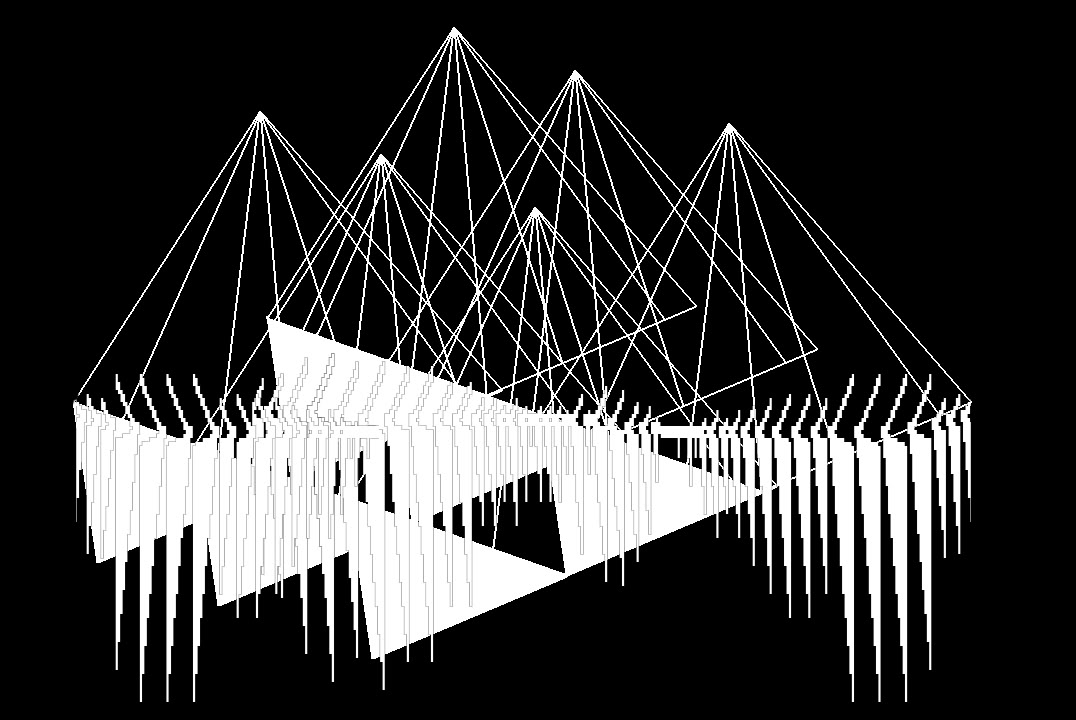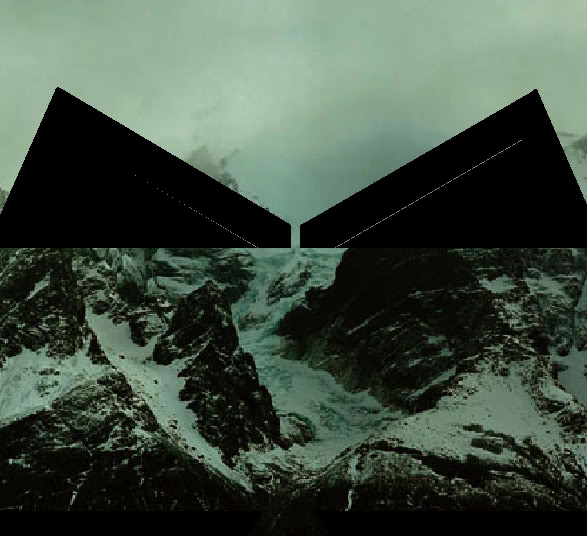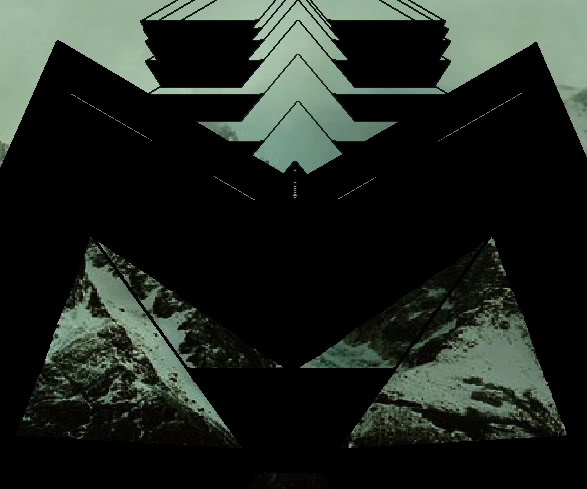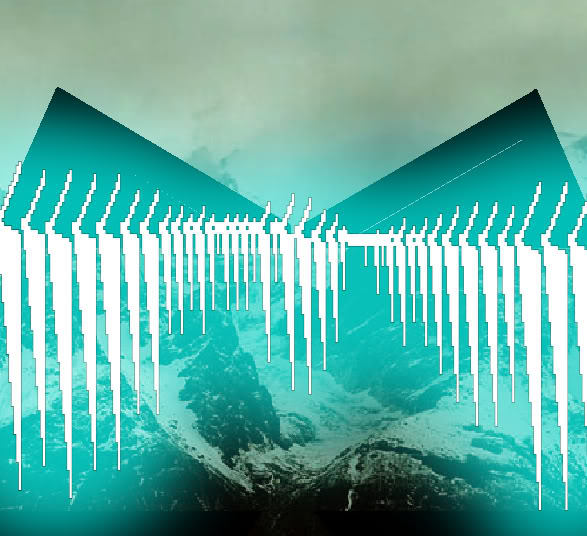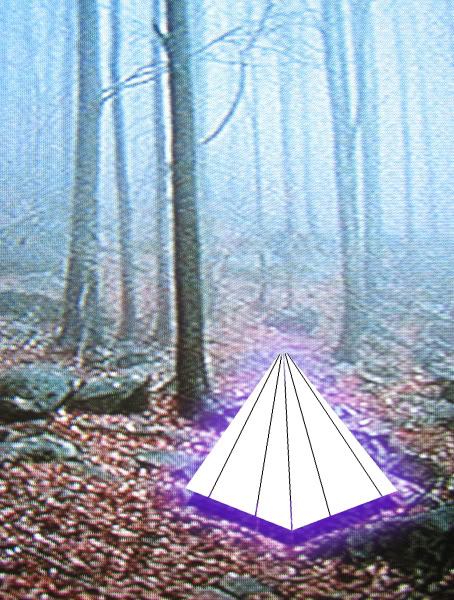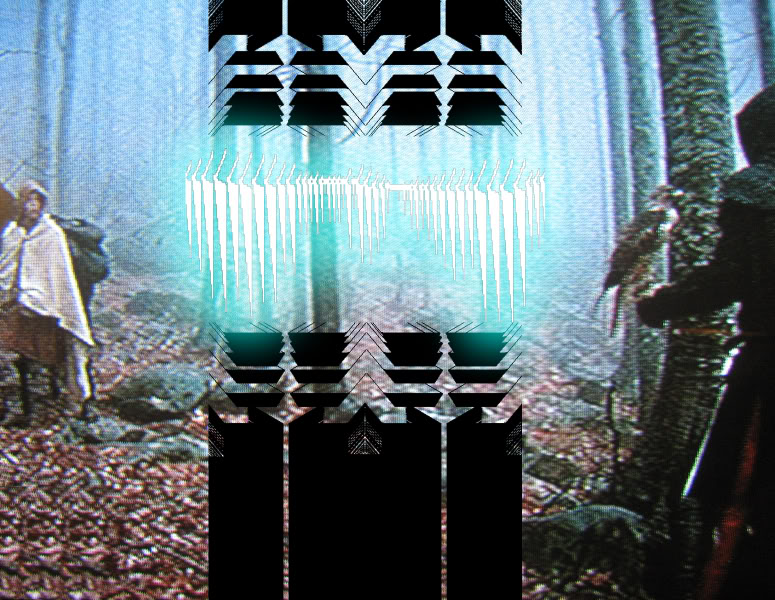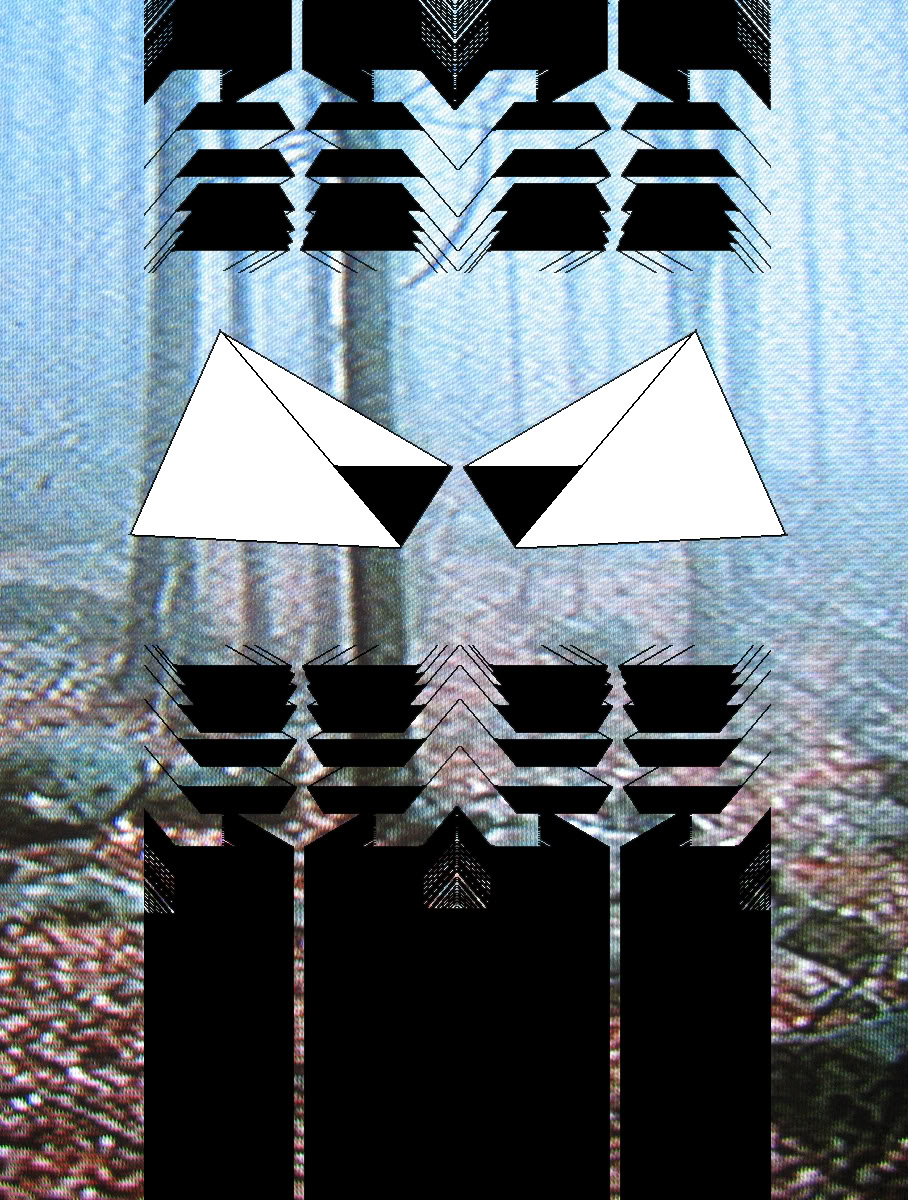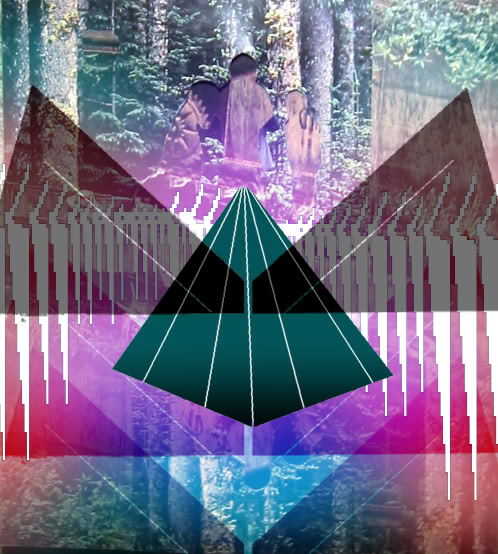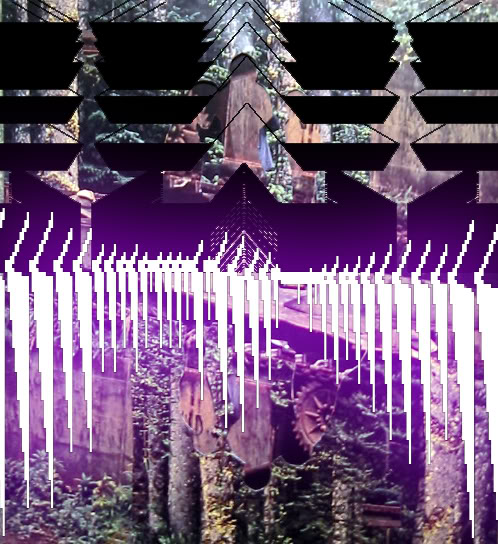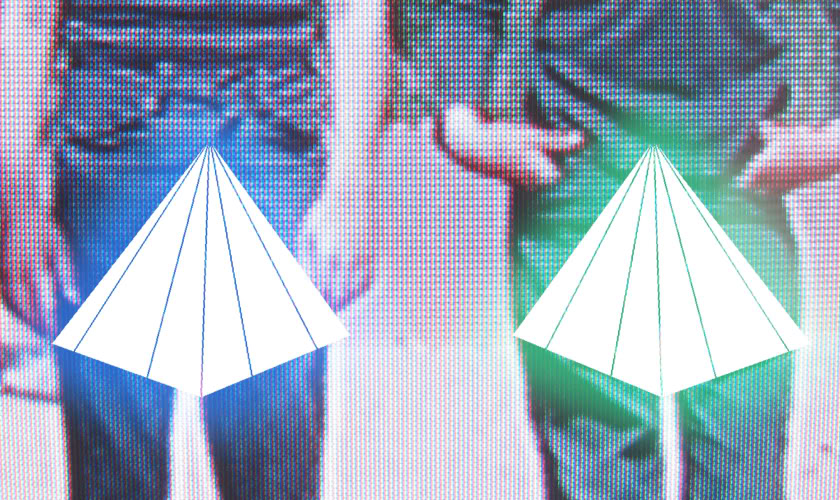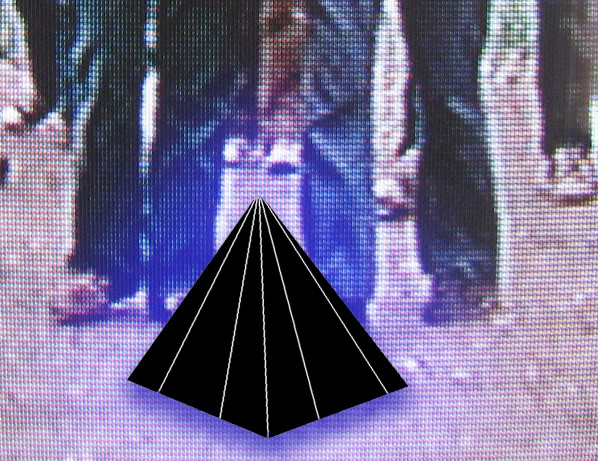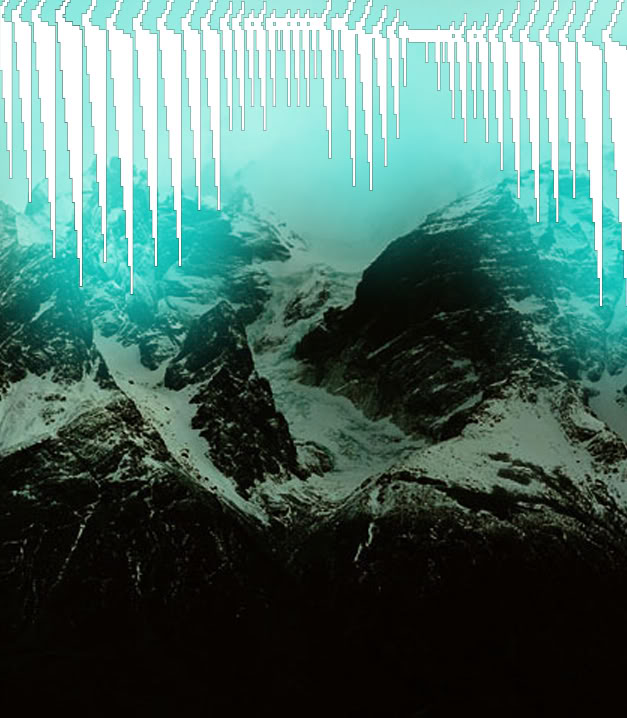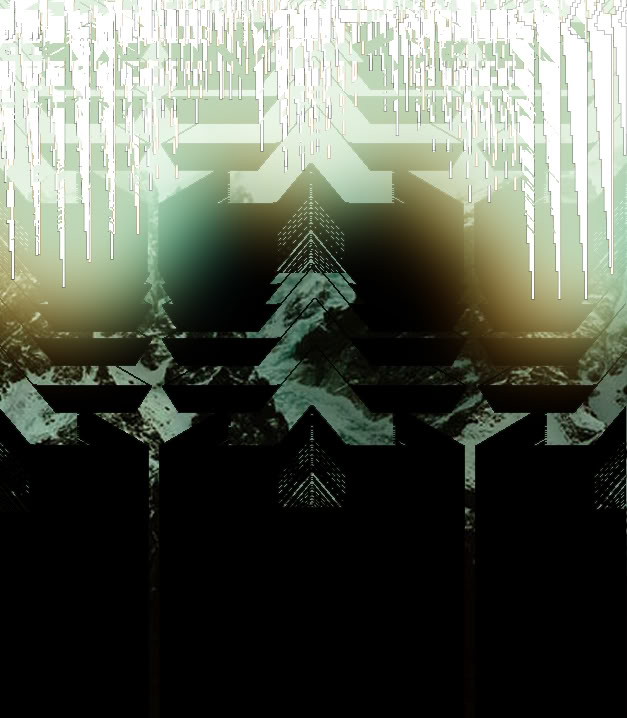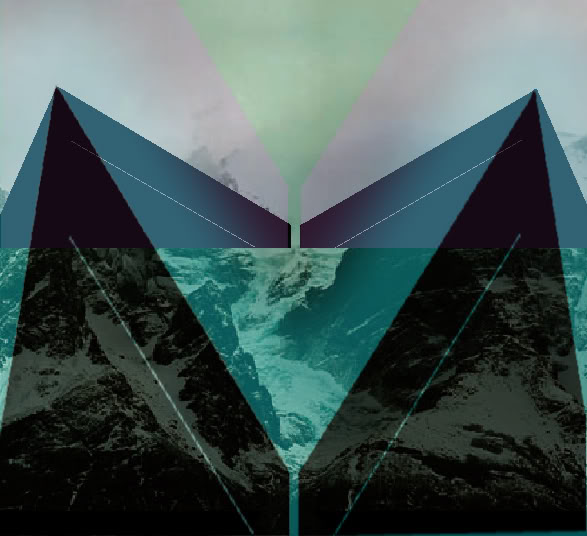a moment like this
𓊍 2007-10-29 23:16:00

ghostbusting with a cloudbuster
𓊍 2007-10-25 13:47:00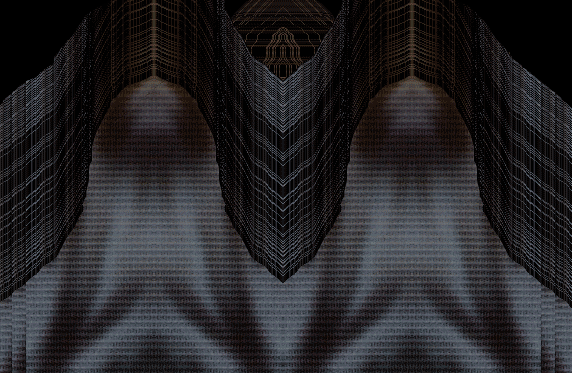diamond soled nikes eat the street
𓊍 2007-10-18 22:24:00

last night's neural noise
𓊍 2007-10-17 23:09:00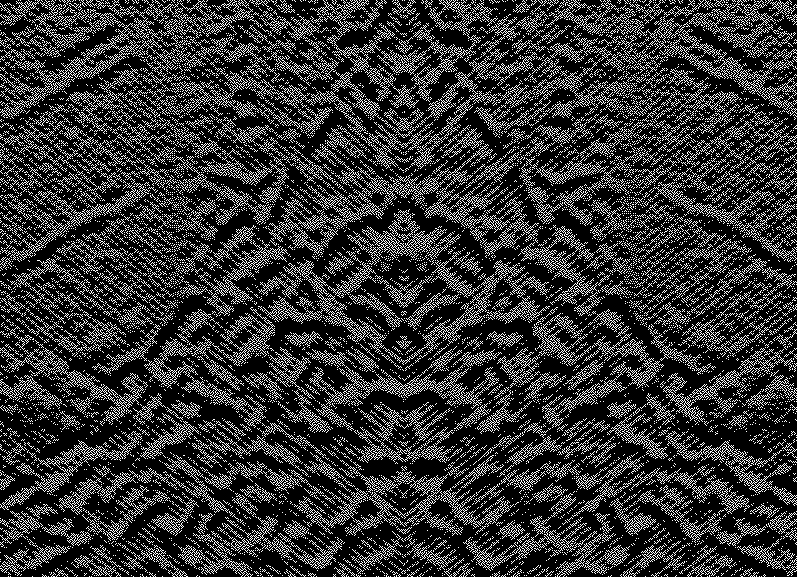nobody's fool
𓊍 2007-10-17 22:46:00

surf^s up
𓊍 2007-10-11 13:57:00

~^~~^~~^~~^~~^~~^~~^~~^~~^~~^~~^~~^~~^~~^~~^~~^~~^~~^~~^~~^~~^~~^~~^~~^~~^~~^~~^~~^~^~~^~~^~~^~~^~~^~~^~~^~~^~~^~~^~~^~~^~~^~~^~~^~~^~~^~~^~~^~~^~~^~~^~~^~~^~~^~~^~~^
~^~~^~~^~~^~~^~~^~~^~~^~~^~~^~~^~~^~~^~~^~~^~~^~~^~~^~~^~~^~~^~~^~~^~~^~~^~~^~~^~~^~^~~^~~^~~^~~^~~^~~^~~^~~^~~^~~^~~^~~^~~^~~^~~^~~^~~^~~^~~^~~^~~^~~^~~^~~^~~^~~^~~^
~^~~^~~^~~^~~^~~^~~^~~^~~^~~^~~^~~^~~^~~^~~^~~^~~^~~^~~^~~^~~^~~^~~^~~^~~^~~^~~^~~^~^~~^~~^~~^~~^~~^~~^~~^~~^~~^~~^~~^~~^~~^~~^~~^~~^~~^~~^~~^~~^~~^~~^~~^~~^~~^~~^~~^
~^~~^~~^~~^~~^~~^~~^~~^~~^~~^~~^~~^~~^~~^~~^~~^~~^~~^~~^~~^~~^~~^~~^~~^~~^~~^~~^~~^~^~~^~~^~~^~~^~~^~~^~~^~~^~~^~~^~~^~~^~~^~~^~~^~~^~~^~~^~~^~~^~~^~~^~~^~~^~~^~~^~~^
~^~~^~~^~~^~~^~~^~~^~~^~~^~~^~~^~~^~~^~~^~~^~~^~~^~~^~~^~~^~~^~~^~~^~~^~~^~~^~~^~~^~^~~^~~^~~^~~^~~^~~^~~^~~^~~^~~^~~^~~^~~^~~^~~^~~^~~^~~^~~^~~^~~^~~^~~^~~^~~^~~^~~^
~^~~^~~^~~^~~^~~^~~^~~^~~^~~^~~^~~^~~^~~^~~^~~^~~^~~^~~^~~^~~^~~^~~^~~^~~^~~^~~^~~^~^~~^~~^~~^~~^~~^~~^~~^~~^~~^~~^~~^~~^~~^~~^~~^~~^~~^~~^~~^~~^~~^~~^~~^~~^~~^~~^~~^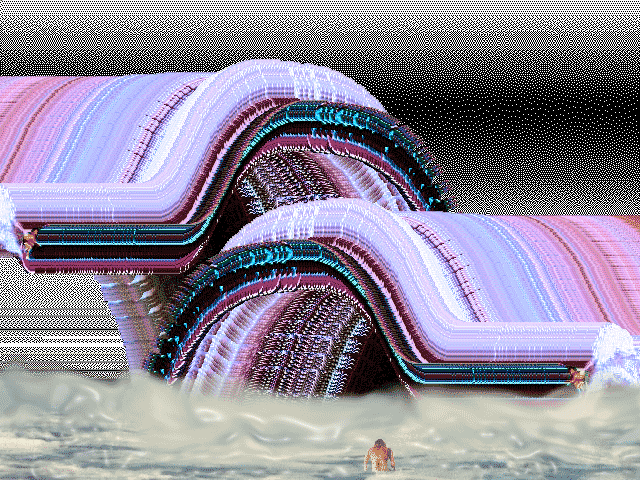~^~~^~~^~~^~~^~~^~~^~~^~~^~~^~~^~~^~~^~~^~~^~~^~~^~~^~~^~~^~~^~~^~~^~~^~~^~~^~~^~~^~^~~^~~^~~^~~^~~^~~^~~^~~^~~^~~^~~^~~^~~^~~^~~^~~^~~^~~^~~^~~^~~^~~^~~^~~^~~^~~^~~^
~^~~^~~^~~^~~^~~^~~^~~^~~^~~^~~^~~^~~^~~^~~^~~^~~^~~^~~^~~^~~^~~^~~^~~^~~^~~^~~^~~^~^~~^~~^~~^~~^~~^~~^~~^~~^~~^~~^~~^~~^~~^~~^~~^~~^~~^~~^~~^~~^~~^~~^~~^~~^~~^~~^~~^
~^~~^~~^~~^~~^~~^~~^~~^~~^~~^~~^~~^~~^~~^~~^~~^~~^~~^~~^~~^~~^~~^~~^~~^~~^~~^~~^~~^~^~~^~~^~~^~~^~~^~~^~~^~~^~~^~~^~~^~~^~~^~~^~~^~~^~~^~~^~~^~~^~~^~~^~~^~~^~~^~~^~~^
~^~~^~~^~~^~~^~~^~~^~~^~~^~~^~~^~~^~~^~~^~~^~~^~~^~~^~~^~~^~~^~~^~~^~~^~~^~~^~~^~~^~^~~^~~^~~^~~^~~^~~^~~^~~^~~^~~^~~^~~^~~^~~^~~^~~^~~^~~^~~^~~^~~^~~^~~^~~^~~^~~^~~^
~^~~^~~^~~^~~^~~^~~^~~^~~^~~^~~^~~^~~^~~^~~^~~^~~^~~^~~^~~^~~^~~^~~^~~^~~^~~^~~^~~^~^~~^~~^~~^~~^~~^~~^~~^~~^~~^~~^~~^~~^~~^~~^~~^~~^~~^~~^~~^~~^~~^~~^~~^~~^~~^~~^~~^
~^~~^~~^~~^~~^~~^~~^~~^~~^~~^~~^~~^~~^~~^~~^~~^~~^~~^~~^~~^~~^~~^~~^~~^~~^~~^~~^~~^~^~~^~~^~~^~~^~~^~~^~~^~~^~~^~~^~~^~~^~~^~~^~~^~~^~~^~~^~~^~~^~~^~~^~~^~~^~~^~~^~~^
~^~~^~~^~~^~~^~~^~~^~~^~~^~~^~~^~~^~~^~~^~~^~~^~~^~~^~~^~~^~~^~~^~~^~~^~~^~~^~~^~~^~^~~^~~^~~^~~^~~^~~^~~^~~^~~^~~^~~^~~^~~^~~^~~^~~^~~^~~^~~^~~^~~^~~^~~^~~^~~^~~^~~^
~^~~^~~^~~^~~^~~^~~^~~^~~^~~^~~^~~^~~^~~^~~^~~^~~^~~^~~^~~^~~^~~^~~^~~^~~^~~^~~^~~^~^~~^~~^~~^~~^~~^~~^~~^~~^~~^~~^~~^~~^~~^~~^~~^~~^~~^~~^~~^~~^~~^~~^~~^~~^~~^~~^~~^
~^~~^~~^~~^~~^~~^~~^~~^~~^~~^~~^~~^~~^~~^~~^~~^~~^~~^~~^~~^~~^~~^~~^~~^~~^~~^~~^~~^~^~~^~~^~~^~~^~~^~~^~~^~~^~~^~~^~~^~~^~~^~~^~~^~~^~~^~~^~~^~~^~~^~~^~~^~~^~~^~~^~~^
~^~~^~~^~~^~~^~~^~~^~~^~~^~~^~~^~~^~~^~~^~~^~~^~~^~~^~~^~~^~~^~~^~~^~~^~~^~~^~~^~~^~^~~^~~^~~^~~^~~^~~^~~^~~^~~^~~^~~^~~^~~^~~^~~^~~^~~^~~^~~^~~^~~^~~^~~^~~^~~^~~^~~^
~^~~^~~^~~^~~^~~^~~^~~^~~^~~^~~^~~^~~^~~^~~^~~^~~^~~^~~^~~^~~^~~^~~^~~^~~^~~^~~^~~^~^~~^~~^~~^~~^~~^~~^~~^~~^~~^~~^~~^~~^~~^~~^~~^~~^~~^~~^~~^~~^~~^~~^~~^~~^~~^~~^~~^
~^~~^~~^~~^~~^~~^~~^~~^~~^~~^~~^~~^~~^~~^~~^~~^~~^~~^~~^~~^~~^~~^~~^~~^~~^~~^~~^~~^~^~~^~~^~~^~~^~~^~~^~~^~~^~~^~~^~~^~~^~~^~~^~~^~~^~~^~~^~~^~~^~~^~~^~~^~~^~~^~~^~~^

too much but never enough
𓊍 2007-10-11 00:19:00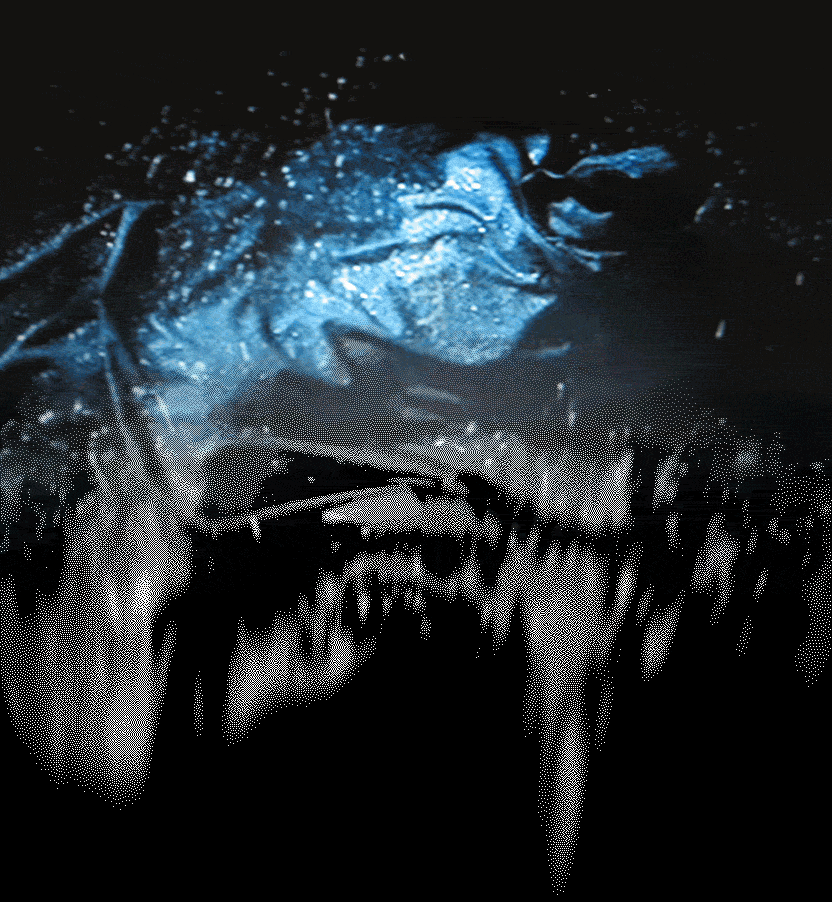rhythm of the nacht moves (the Ambassadors livin by the sword)
𓊍 2007-10-06 21:18:00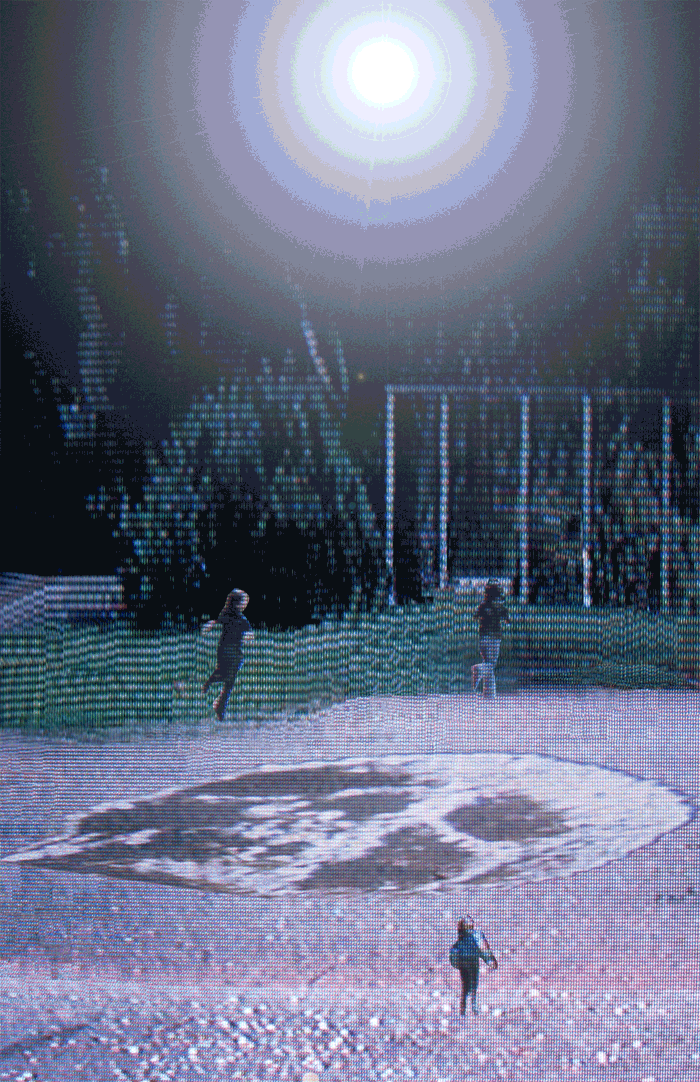moonlit momentary chance to deceive.
𓊍 2007-10-03 21:54:00

encasing the sprawl of splendor
𓊍 2007-09-27 16:46:00